### University of Maryland Physics Education Research Group## Activity Based Physics Thinking Problems in Mechanics: Momentum and Energy

PERG Info | PERG materials | PERG HOMEPAGE | PER on the web | Resources on the web

### Activity Based Physics Thinking Problems in Mechanics: Momentum and Energy

1) The mass of the earth is about 6x1024 kg. Estimate the kinetic energy it has as a result of its orbiting the sun.

2) According to some recent highly accurate measurements made from satellites, the continent of North America is drifting at a rate of about 1 cm per year. Assuming a continent is about 50 km thick, estimate the kinetic energy the continental US has a a result of this motion.

 A bullet of mass m1 is fired with a speed v into the wooden bob of a pendulum having mass m2. The bob is attached to a very light rigid rod of length L that is pivoted at the other end. (a) The bullet is stopped in the bob. How high will the bob rise? (b) A narrower bob with the same mass is used instead of the original one. This time, the bullet passes through the bob, emerging with a speed v/2. Now how high will the bob rise?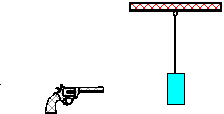3) Two carts on an air track are pushed towards each other. Initially, cart 1 moves in the positive x direction and cart 2 moves in the negative x direction. They bounce off each other elastically. The graphs below describe some of the variables associated with the motion as a function of time. For the experiment described and for each item in the list below, identify which graph is a possible display of that variable as a function of time. If none apply, write N (for none).

(a) the momentum of cart 1
(b) the force on cart 1
(c) the position of cart 2
(d) the force on cart 2
(e) the position of cart 1.4) An electron collides elastically with a hydrogen atom which is initially at rest. The initial and final motions are along the same straight line. What fraction of the electron's initial kinetic energy is transferred to the hydrogen atom? The mass of the hydrogen atom is 1840 times the mass of the electron.

 A block with mass 12.0 kg is being pushed along a horizontal floor by a force P as shown in the following diagram. The kinetic frictional force opposing the motion of the block is constant at a value of 15.0 N. At clock reading t=0.00 s and position x = 0.00 m the block has an instantaneous velocity of 2.00 m/s. The angle is q=0.35 rad. The force P is applied to the block at the instant it is in position x=0.00 m. The direction of P remains fixed, but its magnitude varies with position as shown in the graph at the right. Calculate the various quantities asked for in the following sequence. Use work, kinetic energy, momentum, and impulse arguments throughout. Do NOT make use of Newton's second law. In each case, give a brief explanation of your calculation.(a) Calculate the total work done by the force P in the displacement from position x=0.00 to x=4.00 m. (b) Calculate the work done by the frictional force in the same displacement. (c) Calculate the work done by the net force acting on the block during this displacement. (d) Calculate the change in kinetic energy of the block. (e) Calculate the final kinetic energy of the block, that is, the total kinetic energy it possesses on reaching position x=4.00 m. (f) Calculate how far the block will slide beyond position x=4.00 m if the force P abruptly drops to zero at this position and remains zero from there on. (g) Calculate and describe any internal potential energy changes of the block-earth system that take place over the entire history of the motion dealt with above. (h) Calculate and describe any internal thermal energy changes of the block-floor system over the entire history of the motion dealt with above. (i) Calculate the velocity of the block at position x=4.00 m. (j) Calculate the momentum of the block at position x=4.00 m. (k) Calculate the momentum change imparted to the block over the interval between positions x=0.00 and x=4.00 m. (l) Calculate the net impulse that must have been delivered to the block over this interval.Based on a problem from the book of Arnold Arons.

5) The conservation of momentum is useful in some situations and not in others. Describe how you obtain the impulse-momentum theorem from Newton's second law and what situations lead to momentum conservation. How would you decide if conservation of momentum could be used in a particular problem?

6) Energy conservation is sometimes a useful principle in helping us solve problems concerning the motion of objects. Suppose a single object is moving subject to a number of forces. Describe how you would know whether energy conservation would hold for the given example and in what kinds of problems you might find it appropriate to use it.

7) A professor of physics is going ice skating for the first time. He has gotten himself into the middle of an ice rink and cannot figure out how to make the skates work. Every motion he makes simply slips on the ice and leaves him in the same place he started. He decides that he can get off the ice by throwing his gloves in the opposite direction.

(a) Suppose he has a mass M and his gloves have a mass m. If he throws them as hard as he can away from him, and they leave his hand with a velocity v. Explain whether or not he will move. If he does move, calculate his velocity, V.
(b) Discuss his motion from the point of view of the forces acting on him.
(c) If the ice rink is 10 m in diameter and the skater starts in the center, estimate how long it will take him to reach the edge, assuming there is no friction at all.

8) A child's game consists of a block that attaches to a table with a suction cup, a spring connected to that block, a ball, and a launching ramp. By compressing the spring, the child can launch the ball up the ramp.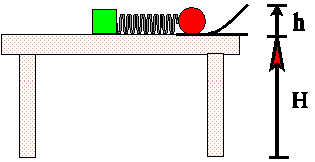The spring has a spring constant k, the ball has a mass m, and the ramp rises a height h. The spring is compressed a distance s in order to launch the ball. When the ball leaves the launching ramp its velocity makes an angle q with respect to the horizontal.

(a) Assuming that friction and air resistance can be ignored for the purposes of this problem, describe the changes in the forms of energy in the system from the time the spring is compressed until the ball first hits the ground.
(b) Calculate the vector velocity of the ball when it leaves the launching ramp. Be sure to specify your coordinate system.
(c) The spring constant = 32 N/m, the spring's compression is 5 cm, the ball's mass is 20 grams, the height of the ramp is 10 cm, and the top of the table is 1 m above the floor. With what speed will the ball hit the floor? (Use g » 10 m/s2.)

9) Two identical carts of mass m are sliding on an air track. Cart 1 approaches cart 2 with velocity V1. Cart 2 is at rest. Cart 1 has a spring on its leading edge. The spring has a spring constant k and is held compressed a distance c by a latch. When cart 1 touches cart 2, the latch is released and all the spring's energy is given to the motion of the two carts. Ignore frictional losses in the spring and to the track.

(a) You want to find the velocities of the two carts after the collision, V1' and V2'. Write two equations which will let you do this and state why you can use them here.
(b) Solve your equations for V1' and V2'. If you find two solutions, discuss which of your solutions you want to use. (Hint: Consider the case when the spring constant goes to 0.) Does the second cart move faster or slower than it would if there were no spring?

10) Construct the Newton drag law for air resistance by filling in the equations that go with the following arguments. Assume a sphere of radius R is moving with velocity v through a dense crowd of tiny particles, of number density n per cm2, with each having mass m.

(a) Assume in a small time Dt that the sphere's velocity doesn't change. What volume of air does it move through and how many particles get swept away in the time Dt?
(b) Assume that each particle "swept away" has its velocity changed from 0 to 2v. What is the total momentum, Dp, added to the air molecules by being "swept away" by the sphere in the time interval Dt?
(c) If the particle hits the molecules and changes their momentum by Dp, it must exert a force on them. By N III, the molecules must also exert a force back on the ball. Use the impulse-momentum theorem to calculate the force on the ball exerted by the air molecules.
(d) Compare your result to the one derived in class and show it has the form |F| = hrR2 v2. What value of h do you get?

11) When objects are too small to be seen by a microscope, physicists often probe them by shooting microscopic particles from the objects and observing how the particles scatter. (R. P. Feynman once described this process as "trying to find out the shape of a wineglass by shooting buckshot at it"!) The M.U.P.P.E.T. program SCATTER * simulates a simple model for this process.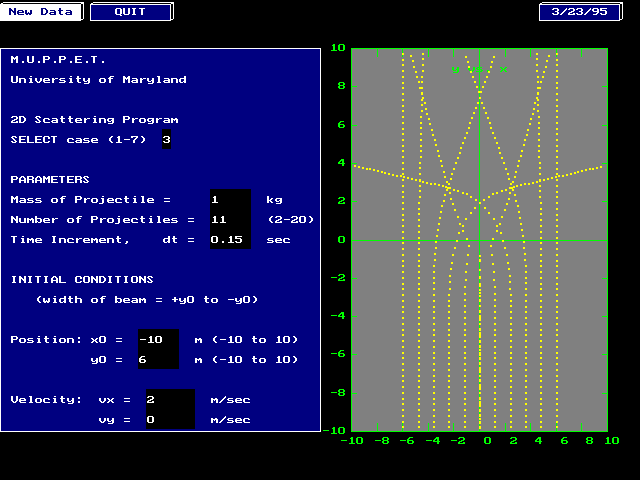The program models shooting a beam of classical particles at a force field in two dimensions. (The best way to think about this is to imagine rolling a bunch of marbles or ball bearings along the ground and trying to use their paths as seen from above to determine the shape of hills and valleys in the ground they move over. The height of the ground corresponds to the potential energy of the force field.) A typical screen is shown below. The particles start at the bottom of the graph and move upward. The picture shown has a beam of 5 particles all travelling parallel toward the top of the graph at a speed of 2 m/s. You can change the number of particles, the width of the beam (by changing y0), and the speed.

The trajectory of each particle is laid down as dots, one place every 0.15 second. (You can change this by changing dt.) When the dots are close together the particle is moving slowly. When they are far apart, the particle is moving fast.

There are 7 different force fields that you may probe. Choose one, find out all you can about it and write up your results. You may give information about the force as a function of position or about the potential energy as a function of position. You may use energy conservation, momentum conservation, or whatever else you like. You may print out the screen and measure the change in spacing of the dots to get the acceleration.

12) An electron at rest has an angular momentum equal to h/4p, where h = Planck's constant (6.63 x 10-34 J-s). At one time it was suggested that the electron was a uniform sphere with radius equal to about 2 x 10-15 m. (This value is obtained by setting the electrostatic potential energy of a uniform sphere of charge equal to mc2. Suppose this were so, and the angular momentum arose from the sphere's rotating with an angular velocity w

(a) Find the value of w required to get the necessary angular momentum.
(b) Calculate the speed with which the electron's equator would be rotating.
(Your answer should come out greater than the speed of light. Rumor has it that in the 1920's one student was discouraged from publishing his idea that the electron had a spin by Wolfgang Pauli presenting him with this calculation. The result is wrong for two reasons. First, if the electron were a sphere spinning that fast, one would have to take relativity into account. The classical formula for angular momentum wouldn't hold. Second, as far as we know, the electron isn't a sphere but is about as close as we can get to a true point. It has built in angular momentum anyhow -- but not from spinning!)

13) An oxygen molecule of mass M is sitting at rest minding its own business. Suddenly, a cosmic ray photon passes by and adds an energy D to the molecule. The molecule sits and wiggles for a while, and the breaks up into two equal mass oxygen atoms traveling in opposite directions.

(a) How fast is each oxygen atom traveling?
(b) Suppose the process took place while the oxygen molecule was traveling with a velocity V and the atoms went out after the breakup in the same direction as V. What velocities would they have viewed from this frame?

14) (a) Describe how a solid ball can move so that (i) its total kinetic energy is just the energy of motion of its center of mass, and (ii) its total kinetic energy is the energy of its motion relative to its center of mass.
(b) Two bowling balls are moving down a bowling alley so that their centers of mass have the same velocity, but one just slides down the alley, while the other rolls down the alley. Which ball has more energy? Explain.

 15) Two carts are riding on an air track as shown in the figure at the right. At clock time t = 0 cart B is at the origin traveling in the +x direction with a velocity speed vB0. At that time, cart A is at the position shown and is at rest. Cart B has twice the mass of cart A. The carts "bump" each other, but don't stick.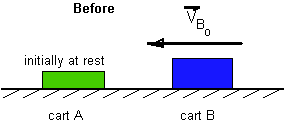In the graphs below are shown a number of possible plots for the various physical parameters associated with the two carts. Each graph has two curves, one for each cart and labeled with the cart's letter. For each property (a)-(e) select the letter of the graph that could be a plot of the property.

(a) The force exerted by the carts.
(b) The position of the carts
(c) The velocity of the carts
(d) The acceleration of the carts
(e) The momentum of the carts.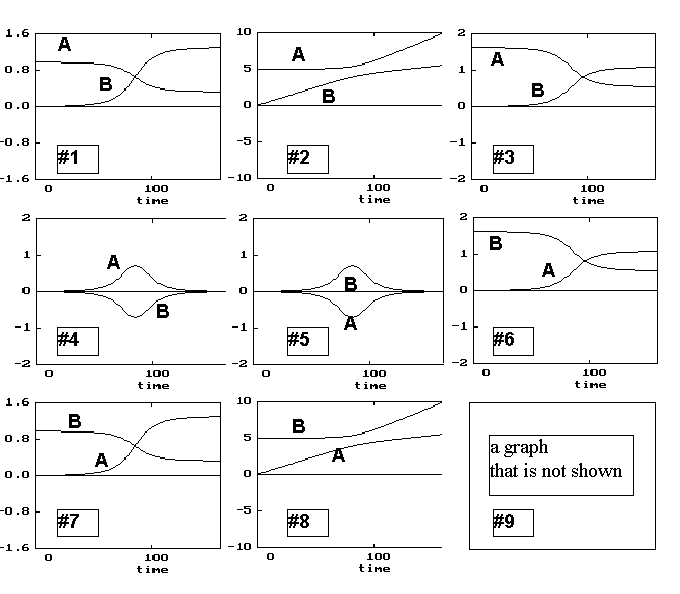16) Can a system whose momentum is conserved be made up of smaller systems whose individual momenta are not conserved? Explain why or why not and give an example.

17) In the figure below are shown two carts on an air track. The carts have equal masses. At the time shown, cart B is moving in the negative x direction and the center of mass of cart A is at the origin and at rest. When the carts collide, they stick together. Friction with the track is small and may be neglectedIn the graphs below are shown a number of possible plots for the various physical parameters associated with one of the two carts. For each property (a)-(e) select the number of the graph that could be a plot of the property as a function of time.

(a) the momentum of cart A
(b) the kinetic energy of cart B
(c) the position of (the center of mass) of cart B
(d) the acceleration of cart A
(e) the total momentum of carts A+B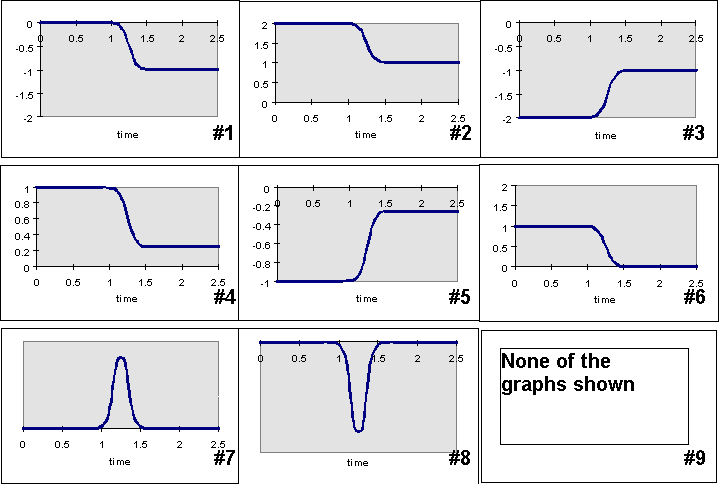18) Energy conservation is sometimes a useful principle in helping us solve problems concerning the motion of objects. Suppose a single object is moving subject to a number of forces. Describing how you would know whether energy conservation would hold for the given example and in what kinds of problems you might find it appropriate to use it.

19) Is it possible for a system of interacting objects to conserve momentum but not mechanical energy (kinetic + potential)? Discuss and defend your answer, then given an example that illustrates the case you are trying to make.

 20) A block, of mass M = 0.50 kg, rests on a frictionless horizontal table so that it is in contact with a spring of negligible mass and spring constant k = 600 N/m. A toy dart, of mass m = 0.10 kg, is shot at the block with a speed vi = 15 m/s (as is shown in part (a) of the figure). The dart sticks to the block with a suction cup. As a result of the impact, the spring first compresses (as shown in part (b) of the figure). After the spring has returned to its equilibrium position, the block and dart move away from the spring, traveling to the left with a speed vf along the table (as shown in part (c) of the figure). (a) What is the change of length of the spring as it is compressed from its initial length to its length at maximum compression? (b) At the instant when the block is momentarily at rest and the spring is at its maximum compression, is the acceleration of the block zero, directed to the right, or directed to the left? Explain why. (c) What is the final speed vf of the block and attached dart? From the book of Fred Reif.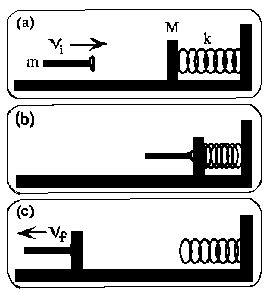21) Two fan carts labeled A and B are placed on opposite sides of a table with their fans pointed in the same direction as shown in the figure below. Cart A is weighted with iron bars so it is twice as heavy as cart B. When the fans are turned on, they provide a constant force of the cart independent of its mass. Assume that friction is small enough to be neglected. The fans are set with a timer so that after they are switched on, they stay on for a fixed length of time, Dt, and then go off.(a) Just after the fans go off, which of the following statements are true about the momenta of the two carts? (i) pA > pB (ii) pA = pB (iii) pA < pB (b) Just after the fans go off, which of the following statements are true about the kinetic energies of the two carts? (i) KA > KB (ii) KA = KB (iii) KA < KB (c) Which of the following statements are true? You may choose as many as you like, or none. If you choose none, write N. (i) After the fan is turned on, each cart moves at a constant velocity, but the two velocities are different from each other. (ii) The kinetic energy of each cart is conserved. (iii) The momentum of each cart is conserved.

22) Kinetic energy and momentum are both quantities that tell something about an object's motion. Define what each one is and discuss the similarities and differences between them.

23) Two blocks collide on a frictionless surface. After the collision the two blocks stick together. Block A has a mass M and is initially moving at speed V. Block B has a mass 2M and is initially at rest. System C is composed of both blocks.a) Draw a free body diagram for each block at an instant during the collision.
b) Rank the magnitudes of the horizontal forces in your diagram. Explain your reasoning.
c) Calculate the change in momentum of block A, block B, and system C. Show all work.
d) Is kinetic energy conserved in this collision? Explain your reasoning.

* To download an executable of the program SCATTER, click on the program name. This program is a DOS program. (It can be run from Windows, but you will probably need to create a PIF file to do so.) To run it you must also have a "Borland Graphics Interface" (BGI) file appropriate for your graphics screen in the same directory as the program. For most computers today, the appropriate file is "EGAVGA.BGI". Both the program and the BGI file are contained in a "zip" file. Unzip them into the same directory using PKUNZIP or WINUNZIP.

These problems written and collected by E. F. Redish. Photos and figures by E. F. Redish. These problems may be freely used in classrooms. They may be copied and cited in published work if the Activity Based Physics Thinking Problems in Physics site is mentioned and the URL given. Web page edited by K. A. Vick.

To contribute problems to this site, send them to redish@physics.umd.edu.

Go back to the Activity Based Physics page
Go back to the Thinking Problems page
Go back to the Thinking Problems in Mechanics page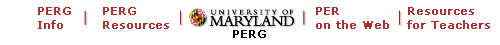Maintained by University of Maryland PERG
Comments and questions may be directed to E. F. Redish